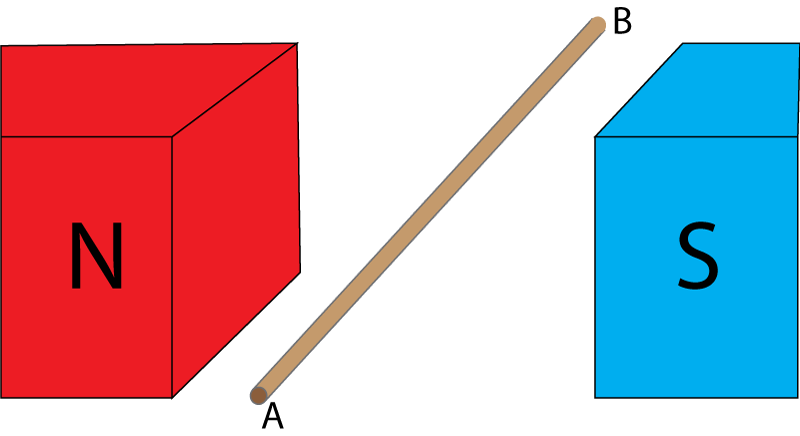10 minutes maximum! Can you do it in 5? 1. A copper wire has a magnetic field around it if... A. it has been magnetised. B. there is a current flowing through it. C. it is twisted into a coil. D. it is heated up. ABCD Q 2-4. The diagram below shows a copper wire between 2 magnets.2. In which direction is the magnetic field? A. N to S B. S to N C. A to B D. B to A ABCD 3. A current flows through the wire from A to B. Which way is the wire pushed? A. To the right B. To the left. C. Upwards. D. Downwards. ABCD 4. If we want the force on the wire to be reversed, which way must current flow? A. N to S B. S to N C. A to B D. B to A ABCD 5. If we want the wire to be pushed to the right, which way must current flow? A. A to B B. B to A C. Use an alternating current. D. This is not possible using a current. ABCD Q6-9. The magnets and wire are re-arranged. A current flows in the wire as show by the arrow.6. Using the left-hand rule, what represents the magnetic field? A. Thumb. B. First finger. C. Second finger. D. Third finger. ABCD 7. In which direction will this wire be pushed? A. To the left. B. To the right. C. Upwards. D. Downwards. ABCD 8. There are several ways to increase the size of the force on the wire. Which of these will not work? A. use a wire with less mass. B. Increase the current. C. Increase the strength of the magnetic field. D. Increase the length of wire in the field. ABCD 9. What could be done to push the wire in the opposite direction, with twice the force? A. Switch the N and S poles of the magnets round. B. Double the current. C. Switch the direction of the current AND the N and S poles. D. Double the current AND switch the N and S poles. ABCD 10. This coil of wire has a current flowing through it which makes the coil move.Which way does the coil move? A. To the left. B. To the right. C. Rotates clockwise. D. Rotates anti-clockwise. ABCD